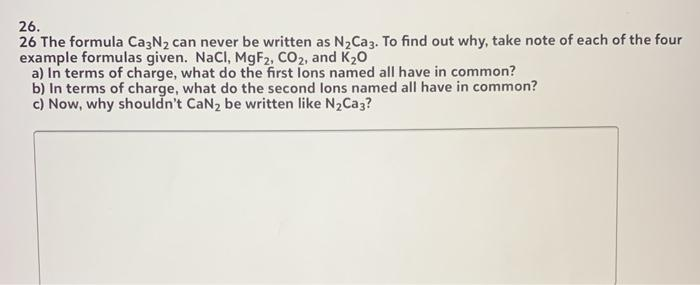Create an Account

Home / Questions / The formula Ca3N2 can never be written as N,Caz. To find out why, take note of each of the...

The formula Ca3N2 can never be written as N,Caz. To find out why, take note of each of the four example formulas given. NaCl, MgF2, CO2, and K20 a) In terms of charge, what do the first lons named all

The formula Ca3N2 can never be written as N,Caz. To find out why, take note of each of the four example formulas given. NaCl, MgF2, CO2, and K20 a) In terms of charge, what do the first lons named all have in common? b) In terms of charge, what do the second lons named all have in common? c) Now, why shouldn't CaNz be written like N2Caz?May 20 2021 View more View LessSubscribe To Get Solution